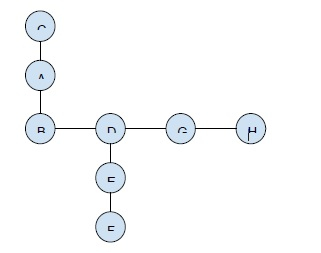# Maximum product of two non-intersecting paths in a tree in C++

C++Server Side ProgrammingProgramming

#### C in Depth: The Complete C Programming Guide for Beginners

45 Lectures 4.5 hours

#### Practical C++: Learn C++ Basics Step by Step

Most Popular

50 Lectures 4.5 hours

#### Master C and Embedded C Programming- Learn as you go

66 Lectures 5.5 hours

In this problem, we are given an undirected connected tree T with n nodes. Our task is to create a program to find the Maximum product of two nonintersecting paths in a tree in C++.

Problem Description − To find the maximum product of two nonintersecting paths in a tree. We will find all the non-interesting paths and then find the product of their lengths.

Let’s take an example to understand the problem,

## Input

Graph −## Output

8

## Explanation

The non-intersecting paths that are considered are C-A-B and F-E-D-G-H.

The lengths are 2 and 4. Product = 8.

## Solution Approach

The solution to this problem is by traversing the tree using DFS. And find the paths that will be unique if we remove the connecting edge. And then iterate on the path and find its length. Then we will pair to paths and find the product of their lengths. The Two are considered in such a way that their product becomes maximum.

Program to implement our solution,

## Example

Live Demo

#include <bits/stdc++.h>
using namespace std;
int TreeTraverse(vector<int> graph[], int& currPathMax, int val1, int val2){
int max1 = 0, max2 = 0, maxVal = 0;
for (int i = 0; i < graph[val1].size(); i++) {
if (graph[val1][i] == val2)
continue;
maxVal = max(maxVal, TreeTraverse(graph, currPathMax,
graph[val1][i], val1));
if (currPathMax > max1) {
max2 = max1;
max1 = currPathMax;
}
else
max2 = max(max2, currPathMax);
}
maxVal = max(maxVal, max1 + max2);
currPathMax = max1 + 1;
return maxVal;
}
int FindMaxProductPath(vector<int> graph[], int Size) {
int maxProd = -10;
int pathA, pathB;
int currPathMax, prod;
for (int i = 0; i < Size; i++) {
for (int j = 0; j < graph[i].size(); j++){
currPathMax = 0;
pathA = TreeTraverse(graph, currPathMax, graph[i][j],i);
currPathMax = 0;
pathB = TreeTraverse(graph, currPathMax, i,graph[i][j]);
prod = (pathA * pathB);
maxProd = max(maxProd, prod);
}
}
return maxProd;
}
void insertEdge(vector<int> graph[], int val1, int val2){
graph[val1].push_back(val2);
graph[val2].push_back(val1);
}
int main(){
int Size = 8;
vector<int> graph[Size + 2];
insertEdge(graph, 1, 2);
insertEdge(graph, 2, 4);
insertEdge(graph, 3, 1);
insertEdge(graph, 5, 4);
insertEdge(graph, 7, 8);
insertEdge(graph, 8, 4);
insertEdge(graph, 5, 6);
cout<<"Maximum product of two non-intersecting paths of tree is "<<FindMaxProductPath(graph, Size)<<"\n";
return 0;
}

## Output

Maximum product of two non-intersecting paths of tree is 8